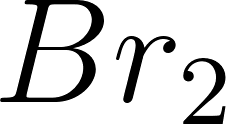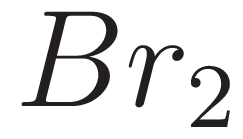×
Get Full Access to Introductory Chemistry - 5 Edition - Chapter 16 - Problem 38p
Get Full Access to Introductory Chemistry - 5 Edition - Chapter 16 - Problem 38p

×

# Solved: For each of the reactions in 36, identify the oxidizing agent and the reducingISBN: 9780321910295 34

## Solution for problem 38P Chapter 16

Introductory Chemistry | 5th Edition

• Textbook Solutions
• 2901 Step-by-step solutions solved by professors and subject experts
• Get 24/7 help from StudySoup virtual teaching assistantsIntroductory Chemistry | 5th Edition

4 5 1 310 Reviews
27
3
Problem 38P

Problem 38P

For each of the reactions in Problem 36, identify the oxidizing agent and the reducing agent.

Problem 1

For each reaction, identify tine substance being oxidized and the substance being reduced.

(a) Mg(s) + Br2(g) → MgBr2(s)

(b) 2 Cr3+(aq) + 3 Mn(s) → 2 Cr(s) + 3 Mn2+ (aq)

(c) 2 H+ (aq) + Ni(s) → H2(g) + Ni2+ (aq)

Step-by-Step Solution:

Problem 38P

For each reaction, identify the substance being oxidized and the substance being reduced.

(a)(b)(c)Step by Step Solution

Step 1 of 3

(a)

Oxidizing agent is a substance that is easily reduced or gains electrons. Those elements that can form anions will serve as good oxidizing agents.

Reducing agents is a substance that is easily oxidized and loses electrons. Those elements that form cations will serve as good reducing agents.

The given reaction is as follows.“Mg” is oxidized by loose electrons . Hence,it is a reducing agent.reduced by accepting electrons. Hence, it is an oxidizing agent.

Therefore, “Mg” is a reducing agent andis an oxidizing agent.

___________________________________________________________________________

Step 2 of 3

Step 3 of 3

##### ISBN: 9780321910295

This textbook survival guide was created for the textbook: Introductory Chemistry, edition: 5. The answer to “For each of the reactions in 36, identify the oxidizing agent and the reducing agent. For each reaction, identify tine substance being oxidized and the substance being reduced.(a) Mg(s) + Br2(g) ? MgBr2(s)(b) 2 Cr3+(aq) + 3 Mn(s) ? 2 Cr(s) + 3 Mn2+ (aq)(c) 2 H+ (aq) + Ni(s) ? H2(g) + Ni2+ (aq)” is broken down into a number of easy to follow steps, and 55 words. Since the solution to 38P from 16 chapter was answered, more than 443 students have viewed the full step-by-step answer. The full step-by-step solution to problem: 38P from chapter: 16 was answered by , our top Chemistry solution expert on 05/06/17, 06:45PM. This full solution covers the following key subjects: agent, Being, identify, substance, reaction. This expansive textbook survival guide covers 19 chapters, and 2046 solutions. Introductory Chemistry was written by and is associated to the ISBN: 9780321910295.

Unlock Textbook Solution